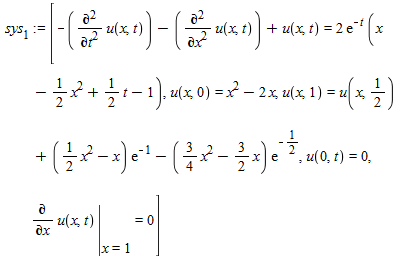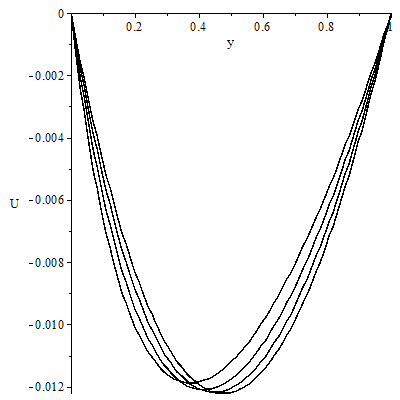## How to import and manipulate xl file in maple...

Hi,

How to import and manipulate different formulas of  Excel files in maple ?

See example in attachment

Thanks

## New special matrix form...

Hi all

I wnat to produce following matrix P2r+1, 2r+1 in maple.

can any one help me please?## Error, (in dsolve/numeric/process_input) system mu...

Error, (in dsolve/numeric/process_input) system must be entered as a set/list of expressions/equations

## do not show answer ...

solve does not show result!!

dtm.mw

## procedure for the solution...

can i have step by step procedure of the solution of the follwoing problem

sys := [-(diff(u(x, t), t, t))-(diff(u(x, t), x, x))+u(x, t) = 2*exp(-t)*(x-(1/2)*x^2+(1/2)*t-1), u(x, 0) = x^2-2*x, u(x, 1) = u(x, 1/2)+((1/2)*x^2-x)*exp(-1)-((3/4)*x^2-(3/2)*x)*exp(-1/2), u(0, t) = 0, eval(diff(u(x, t), x), x = 1) = 0]## How do I use Gram-Schmidt Orthogonalisation to fin...

I am attempting to use the Gram-Schmidt process with Maple to show that the first six orthogonal polynomials which satisfy the following orthogonality condition:

$\int_0^1 (1-x)^{3/2} \phi_n(x) \phi_m(x) dx = h_{n} \delta_{nm}$

can be expressed in the form:

\phi_0(x) = 1, \phi_1(x) = x − 2/7 , \phi_2(x) = x^2 − (8/11)x + 8/99 , \phi_3(x) = x^3 − (6/5)x^2 + (24/65)x − 16/715 , \phi_4(x) = x^4 − (32/19)x^3 + (288/323)x^2 − (256/1615)x + 128/20995 , \phi_5(x) = x^5 − (50/23)x^4 + (800/483)x^3 − (1600/3059)x^2 + (3200/52003)x − 256/156009.

At the same time I have to find the corresponding values for h_n, so for example, h_0 = 2/5 and h_1 = 8/441.  The polynomials which I obtain have to be combined with the Gaussian quadrature method to show that

$\int_0^1 (1-x)^{3/2} \phi_n(x) \phi_m(x) dx = h_{n} \delta_{nm} \approx \sum_{k=1}^4 c_k f(x_k)$

where x_k are the four roots of \phi_4(x)=0 such that x = [0.0524512, 0.256285, 0.548299, 0.827175]

and the 4 c_k coefficients are given by c = [0.121979, 0.168886, 0.0920439, 0.0170909].

I have learned about Gram-Schmidt orthogonalisation in a basic setting in linear algebra courses where a system of N linearly independent orthogonal vectors is constructed from a system of N linearly independent vectors, but unsure how to apply it to polynomials.  I am also vaguely familar with the idea of appoximating integrals with sets of orthogonal polynomials (Legendre, for example) but not exactly sure how this all works.

## System of ODEs taking very long to solve ...

Hi, I'm very new to maple and I'm trying to solve  a system of ODEs but as of now it has taken over 5 hours to solve and is still evaluating. i feel as though I may have made some basic mistakes in the code which make the calculation much longer than it should be. I will try tyo explain the problem as well as I can

I'm trying to model a chemical reaction, and solve for the concentrations of two species involved in the reaction after a given time.

The rate of the reaction is given as: r=2900exp(-53300/RT)*Cno^0.62*Cnh3^-0.05;  where R is a constant, T is temperature and Cno and Cnh3 are the concentrations of NO and NH3 respectively. I am interested in solving for Cno and Cnh3 and getting an expression for each of them. I have tried to set up the system of ODEs as follows:

with(LinearAlgebra):

with(DEtools):

r := 2900*(exp(1)^(-53300/R*T)*CNO(t)^0.62*CNH3(t)^-0.05:

ode := diff(CNO(t), t) = -1* r: (negative because they are decaying with time)

ode2 := diff(CNH3(t), t) = -1* r:

ics := CNO(1020) = 1.6, CNH3(1020) = 1.6; (sets up known initial conditions)

ode := subs(R = 8.314, T = 473, ode):

ode2 := subs(R = 8.314, T = 473, ode2):

sys_ode := (ode, ode2) :

dsolve([sys_ode, ics]);

I wonder if the problem has to do with the boundary conditions that I've set or not. Please help as I know I may have set it up very inefficiently which might be causing the problems. Thank you for your help

## Fermionic Operators on a Lattice...

Using the Physics package (the newest updates for Maple 2016), I am trying to get a basic setup of fermions on a lattice. My lattice is indexed j=1,...,L for some length, L (I'm trying just for L=4 by hand). Using combinat and arraytools and lsit tools, I can generate my entire list of configurations from [0,0,0,..,0], [1,0,0,...,0], ..., [1,1,...,1]. I read through this https://www.mapleprimes.com/questions/151320-Creation-And-Annihilation-Operators# which should be even more general than my problem, but I'm not getting the anti-commutation relationships to work out properly. In that link, the original poster is putting the kets in by hand, I would like to generate them.

So I have this:

restart with(Physics): with(combinat): with(LinearAlgebra): with(ArrayTools): with(ListTools):

Setup(mathematicalnotation=true): Setup(quantumop={cre,ann}, algebrarules={%AntiCommutator(cre[j],ann[k])=I*KroneckerDelta[j,k],%AntiCommutator(cre[j],cre[k])=0, %AntiCommutator(ann[j],ann[k])=0})

and my algebra is defined. But if I try to compute AntiCommutator(ann,ann) is doesn't evaluate it but computes AntiCommutator(cre,ann) perfectly fine.

Okay. The algebra is defined, then I create my configurations by using a traingular array and then permutations (and some list/array tools to product vectors that look like [0,0,0,0],[0,0,0,1],...,[1,1,1,1])

Config_Gen := proc(Length) global Configurations; local fermionarray,n_n,V,Ln,i_i; fermionarray:= Array(triangular[upper],1..Length+1,1..Length,fill=1); for n_n from 1 to Length+1 do V[n_n] := fermionarray(n_n,1..Length); Ln[n_n] := permute(convert(V[n_n],list)); end do; Configurations := Ln; for i_i from 2 to Length+1 do     Configurations := [op( Configurations), op(Ln[i_i] ) ]; #    Configurations := Reverse([op( Configurations), op(Ln[i_i] ) ]); end do;  print("Table of configuratons is", fermionarray);  print("The table written as a set of vectors is", V);  print("Permutations of generators is", Ln);  print("Full list of Configurations is", Configurations); end proc;

For Config_Gen(4) this makes the configurations perfectly fine. Then I need to translate these configurations into creation operators acting on the 0-particle vacuum:

for i_i from 1 to 2^4 do Ket(psi,Configurations[i_i]):= product(cre[j_j]^Configurations[i_i][j_j],j_j = 1 .. 4)* Ket(psi,Vacuum); nnn:= sum(Configurations[i_i][j_j],j_j=1..4) : fff:=(-1)^(nnn*(nnn-1)/2): Bra(psi,Configurations[i_i]):= fff* Bra(psi,Vacuum) * product(( ann[j_j] )^Configurations[i_i][j_j],j_j = 1 .. 4); #[seq(ann,j=1..4)]; end do; value(Simplify(Bra(psi,[1,1,0,1]) *Ket(psi,[1,1,0,1]) ));

Which generates the bras and kets. The factors of (-1) are chosen to give the correct bracket. A problem: For some reason, the product in Maple gives the same when I do Configurations[i_i][(4+1)-j_j].

Some other problems: When I go to evaluate AntiCommutator(ann,ann) or more explicitly ann*ann + ann*ann, the worksheet doesn't simplify. Is there a nice way to use the built in Annihilation and Creation operators of the physics package into the tensorproduct space on which I'd like to do calculations?

## Issues with eps exported files...

Hello,

Here is the code I used to generate the image:

densityplot(x,x=0..1,y=-0..0.1,colorstyle=HUE,style=patchnogrid,scaling=constrained,view=[0..1,0..0.1],axes=none);

Then, I exported the image in a eps format. When the file is displayed, white lines appear as a grid. The problem comes from Maple and not from the softwares used to visualize the image. I tried so many things to solve that but...How to solve the problem?

Thank you.

Jaqr

## How to change odeplot color...

how to change the ode polt color for the below code

with(plots);

R1 := .1; R0 := .1; m := .1; a := .1; Ha := .1; Nt := .1; Nb := .1; Pr := 6.2; Le := .6; Bi := 1; Ec := .1; k := 1; r := .1; A := 1;

fcns := {C(y), T(y), U(y), W(y)};

sys := diff(U(y), $(y, 2))+(R1*(diff(U(y), y))-2*R0*W(y))*exp(a*T(y))-a*(diff(U(y), y))*(diff(T(y), y))-Ha = 0, diff(W(y), $(y, 2))+(R1*(diff(W(y), y))+2*R0*U(y))*exp(a*T(y))-a*(diff(W(y), y))*(diff(T(y), y))-Ha = 0, diff(T(y), $(y, 2))+R1*Pr*(diff(T(y), y))+Pr*Ec*exp(-a*T(y))*((diff(U(y), y))*(diff(U(y), y))+(diff(W(y), y))*(diff(W(y), y)))+Pr*Ha*Ec = 0, diff(C(y), $(y, 2))+Pr*Le*R1*(diff(C(y), y))+Nt*(diff(C(y), \$(y, 2)))/Nb = 0;

bc := U(0) = 0, W(0) = 0, C(0) = 0, (D(T))(0) = Bi*(T(0)-1), U(1) = 0, W(1) = 0, C(1) = 1, T(1) = 0;

L := [.5, 1.0, 1.5, 2.0]; AP := NULL;

for k to 4 do R := dsolve(eval({bc, sys}, Ha = L[k]), fcns, type = numeric, AP); AP := approxsoln = R; p1u[k] := odeplot(R, [y, U(y)], 0 .. 1, numpoints = 100, labels = ["y", "U"], style = line, color = ["black", "blue", "red", "pink"]) end do;

display({p1u, p1u, p1u, p1u})## How to see details of computations of numeric inte...

I have a multiple integration. I know my integrals exist. When I write procedure for Rimannian sum, Maple gives correct answers for every choice of parameters of my integral. But when I ask Maple to compute it with int(...,numeric) sometimes it shows a correct answer and sometimes it shows a wrong answer. I'm curios to know what is going wrong in Maple, is there any command to see the details and steps of the computation to see where Maple is going wrong? I also increased the number of digits after decimal from 10 to 12 and 14. But Maple just repeats the wrong answers with just two or four digits. So the problem is not related to the approximation arising from number of decimals.

## Why maple does not respond to the commands?...

I was calculating the total derivative of a function but maple does not respond to the commands like alias,diff,totaldiff etc.

fsolve.mw

## Heatmap Matrix all values +1 or -1, try with Spar...

Hello, I want to repeat in matrix for two colors a matrix, if the element i, j
takes the value +1 is blue and if it is -1 green, it happens that sometimes
the matrix can take all the values ​​as +1 or -1, I used heatmap, but apparently
it gives problems if all the elements of the matrix take +1 or -1.

restart;
sizeMatriz := 4; iter := 1000; with(Statistics); with(LinearAlgebra); randomize(); with(plots);
sizeMatriz := 4
iter := 1000
f := rand(1 .. 2);
f := proc()  ...  end;
g := rand(1 .. 10);
g := proc()  ...  end;
k := 1;
k := 1
RM := Matrix(sizeMatriz, sizeMatriz, proc (i, j) options operator, arrow; 1 end proc); HeatMap(RM, axis = [gridlines = [sizeMatriz, color = green], thickness = 2], color = [blue, yellow], size = [640, 480]);
Error, (in Statistics:-HeatMap) the 2nd argument should be of type {numeric, string}

How i made this?, is posible by other command that not Heatmap, other way? I try with sparsematrix:

restart;
sizeMatriz := 4; iter := 1000; with(Statistics); with(LinearAlgebra); randomize(); with(plots);
sizeMatriz := 4
iter := 1000
f := rand(1 .. 2);
RM := Matrix(sizeMatriz, sizeMatriz, proc (i, j) options operator, arrow; (-1)^f() end proc)
PA := sparsematrixplot(RM, matrixview, color = ["Blue","Red"]);

but this paint alls vaues matrix[i,j] +1 or -1 in the same color.

Regards.

## Simplification of an expression...

Dear users!

Hope everyone should be fine here. I need the following simiplification. I did it step by step is there and maple command to do this.

(diff(theta(eta), eta, eta))*(Rd*T[infinity]^3*(&theta;w-1)^3*theta(eta)^3+3*Rd*T[infinity]^3*(&theta;w-1)^2*theta(eta)^2+(3*(Rd*T[infinity]^3+(1/3)*epsilon*k[nf]))*(&theta;w-1)*theta(eta)+Rd*T[infinity]^3+k[nf]) = (-3*Rd*T[infinity]^3*(&theta;w-1)^3*theta(eta)^2-6*Rd*T[infinity]^3*(&theta;w-1)^2*theta(eta)+(-3*Rd*T[infinity]^3-epsilon*k[nf])*(&theta;w-1))*(diff(theta(eta), eta))^2+(-(rho*c[p])[nf]*nu[f]*f(eta)-(rho*c[p])[nf]*nu[f]*g(eta))*(diff(theta(eta), eta))+a*nu[f]*mu[nf]*(diff(f(eta), eta))^2/((-&theta;w+1)*T[infinity])-2*a*nu[f]*mu[nf]*(diff(g(eta), eta))*(diff(f(eta), eta))/((&theta;w-1)*T[infinity])+a*nu[f]*mu[nf]*(diff(g(eta), eta))^2/((-&theta;w+1)*T[infinity])
(diff(theta(eta), eta, eta))*Rd*T[infinity]^3*(theta(eta)*&theta;w-theta(eta)+1)^3+(diff(theta(eta), eta, eta))*k[nf]*(epsilon*theta(eta)*&theta;w-epsilon*theta(eta)+1)+3*Rd*T[infinity]^3*(&theta;w-1)*(theta(eta)*&theta;w-theta(eta)+1)^2*(diff(theta(eta), eta))^2+epsilon*k[nf]*(&theta;w-1)*(diff(theta(eta), eta))^2
diff((theta(eta)*&theta;w-theta(eta)+1)^3*(diff(theta(eta), eta))*Rd*T[infinity]^3, eta)+diff((epsilon*theta(eta)*&theta;w-epsilon*theta(eta)+1)*(diff(theta(eta), eta))*k[nf], eta);